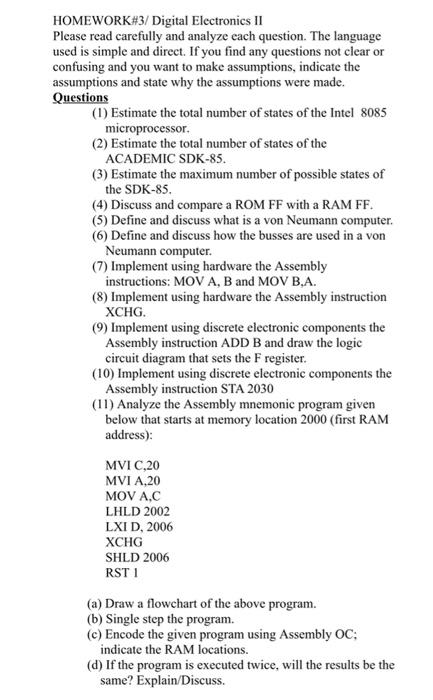# Question help asap HOMEWORK#3/ Digital Electronics II Please read carefully and analyze each question. The language used is simple and direct. If you find any questions not clear or confusing and you want to make assumptions, indicate the assumptions and state why the assumptions were made. Questions (1) Estimate the total number of states of the Intel 8085 microprocessor (2) Estimate the total number of states of the ACADEMIC SDK-85. (3) Estimate the maximum number of possible states of the SDK-85. (4) Discuss and compare a ROM FF with a RAM FF. (5) Define and discuss what is a von Neumann computer. (6) Define and discuss how the busses are used in a von Neumann computer. (7) Implement using hardware the Assembly instructions: MOV A, B and MOV B.A. (8) Implement using hardware the Assembly instruction XCHG, (9) Implement using discrete electronic components the Assembly instruction ADD B and draw the logic circuit diagram that sets the F register. (10) Implement using discrete electronic components the Assembly instruction STA 2030 (11) Analyze the Assembly mnemonic program given below that starts at memory location 2000 (first RAM address): MVI C,20 MVI A.20 MOV A.C LHLD 2002 LXID, 2006 XCHG SHLD 2006 RSTI (a) Draw a flowchart of the above program. (b) Single step the program. (c) Encode the given program using Assembly OC; indicate the RAM locations. (d) If the program is executed twice, will the results be the same? Explain/DiscussJNHPY7 The Asker · Electrical Engineering
help asapTranscribed Image Text: HOMEWORK#3/ Digital Electronics II Please read carefully and analyze each question. The language used is simple and direct. If you find any questions not clear or confusing and you want to make assumptions, indicate the assumptions and state why the assumptions were made. Questions (1) Estimate the total number of states of the Intel 8085 microprocessor (2) Estimate the total number of states of the ACADEMIC SDK-85. (3) Estimate the maximum number of possible states of the SDK-85. (4) Discuss and compare a ROM FF with a RAM FF. (5) Define and discuss what is a von Neumann computer. (6) Define and discuss how the busses are used in a von Neumann computer. (7) Implement using hardware the Assembly instructions: MOV A, B and MOV B.A. (8) Implement using hardware the Assembly instruction XCHG, (9) Implement using discrete electronic components the Assembly instruction ADD B and draw the logic circuit diagram that sets the F register. (10) Implement using discrete electronic components the Assembly instruction STA 2030 (11) Analyze the Assembly mnemonic program given below that starts at memory location 2000 (first RAM address): MVI C,20 MVI A.20 MOV A.C LHLD 2002 LXID, 2006 XCHG SHLD 2006 RSTI (a) Draw a flowchart of the above program. (b) Single step the program. (c) Encode the given program using Assembly OC; indicate the RAM locations. (d) If the program is executed twice, will the results be the same? Explain/Discuss
More
Transcribed Image Text: HOMEWORK#3/ Digital Electronics II Please read carefully and analyze each question. The language used is simple and direct. If you find any questions not clear or confusing and you want to make assumptions, indicate the assumptions and state why the assumptions were made. Questions (1) Estimate the total number of states of the Intel 8085 microprocessor (2) Estimate the total number of states of the ACADEMIC SDK-85. (3) Estimate the maximum number of possible states of the SDK-85. (4) Discuss and compare a ROM FF with a RAM FF. (5) Define and discuss what is a von Neumann computer. (6) Define and discuss how the busses are used in a von Neumann computer. (7) Implement using hardware the Assembly instructions: MOV A, B and MOV B.A. (8) Implement using hardware the Assembly instruction XCHG, (9) Implement using discrete electronic components the Assembly instruction ADD B and draw the logic circuit diagram that sets the F register. (10) Implement using discrete electronic components the Assembly instruction STA 2030 (11) Analyze the Assembly mnemonic program given below that starts at memory location 2000 (first RAM address): MVI C,20 MVI A.20 MOV A.C LHLD 2002 LXID, 2006 XCHG SHLD 2006 RSTI (a) Draw a flowchart of the above program. (b) Single step the program. (c) Encode the given program using Assembly OC; indicate the RAM locations. (d) If the program is executed twice, will the results be the same? Explain/Discuss